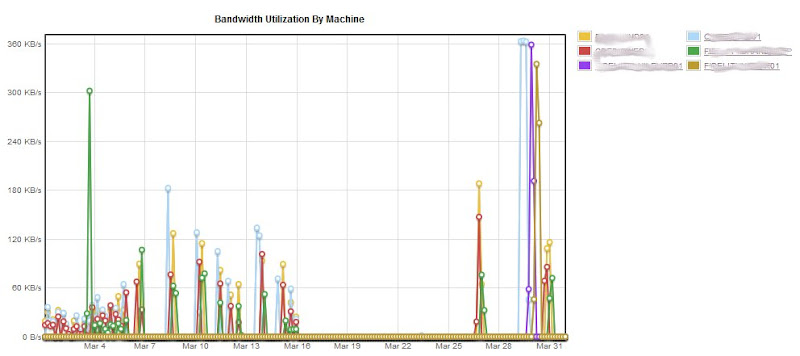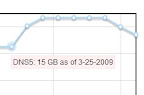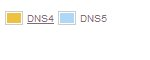Overview

Adding graphs to web pages used to require building up images server side or using a flash applet. Server side images had bandwidth concerns and limited interactivity while flash applets required a another skill set to develop.

With the advent of client side JavaScript libraries like jQuery, several graphing toolkits have been written to allow client side graph generation and interaction. The one we use at work is called flot . It is open source, easy to develop with, provides excellent cross browser functionality, is well integrated with our primary JavaScript library (jQuery) and generates very attractive graphs.Figure 1 - Sample Flot Chart

Flot provides a host of useful features, including:

• Intelligent scaling of axis based on series data
• Zooming and drill down with clickable data points
• Automatically legend creation
• Complete control over formatting, with sensible defaults
• Simple binding to JSON data for interacting with web services
• Interpolation of data. Data sets do not need to have a uniform collection of x-axis values.
• A variety of graph types, including time series, scatter plot, bar, and stacked series. Different graph types can be combined into a single graphFigure 2 - Combining Chart Types

Walkthrough of Simple Graph

Our typical usage for flot is to calculate the data set server side and return a set of data series to load into flot. Flot expects the data for a series to be a array of point values, each point value being a simple two element array. This is then contained inside another object, with additional properties describing the series. We have a collection of classes we use to load the data that serialize well into JSON and that can be fed directly into our flot graph using the flot series format. A simple data series object is show below:

`   1:  public class SimpleDataSeries`

`   2:  {`

`   3:      #region Properties`

`   4:      public string label`

`   5:      {`

`   6:          get { return m_label; }`

`   7:          set { m_label = value; }`

`   8:      }`

`   9:   `

`  10:      private string m_label;`

`  11:   `

`  12:      public List<double[]> data`

`  13:      {`

`  14:      get { return m_data; }`

`  15:      set { m_data = value; }`

`  16:      }`

`  17:   `

`  18:      private List<double[]> m_data;`

`  19:      #endregion`

`  20:   `

`  21:      public SimpleDataSeries(string label)`

`  22:      {`

`  23:            m_label = label;`

`  24:            m_data = new List<double[]>();`

`  25:      }`

`  26:   `

`  27:      public void AddDataPoint(double x, double y)`

`  28:      {`

`  29:            data.Add(new double[] { x, y });`

`  30:      }`

`  31:  }`

Flot has automatic capabilities for displaying and formatting time series data. For time series charts it expects the x values to be in JavaScript timestamps, which are the number of seconds since 1/1/1970. To handle time series we use another server side class:

`   1:  public class TimeDataSeries : SimpleDataSeries`

`   2:  {`

`   3:      #region Properties`

`   4:   `

`   5:      private DateTime UnixStart`

`   6:      {`

`   7:      get { return m_UnixStart; }`

`   8:      set { m_UnixStart = value; }`

`   9:      }`

`  10:      private DateTime m_UnixStart;`

`  11:   `

`  12:      #endregion`

`  13:      public TimeDataSeries(string Label)`

`  14:      :base(Label)`

`  15:      {`

`  16:      UnixStart = new DateTime(1970, 1, 1);`

`  17:      }`

`  18:   `

`  19:      public void AddDataPoint(DateTime x, double y)`

`  20:      {`

`  21:      double JavascriptTimestamp = x.Subtract(UnixStart).TotalMilliseconds;`

`  22:      AddDataPoint(JavascriptTimestamp, y);`

`  23:      }`

`  24:  }`

In order to get this data to the client, we use a web service that returns a listing of these data series in the response object. Here is a web service call that returns a set of data series describing the storage utilization of several servers on our network, both for each machine individually and as a set of summary series:

`   1:  [WebMethod(true)]`

`   2:  public IUpdateResponse Update(IUpdateRequest request)`

`   3:  {`

`   4:      // get the current user`

`   5:      User CurrentUser = User.GetUser(HttpContext.Current.User.Identity.Name);`

`   6:      // create a response and initialize it with base reponse data`

`   7:      UpdateResponse response = new UpdateResponse();`

`   8:      // add our main response properties`

`   9:      VaultStorageCalculator storagecalc = new VaultStorageCalculator(request.VaultId, request.MachineIds, request.StartDate, request.EndDate, 45);`

`  10:   `

`  11:      GraphData gd = storagecalc.GetGraphData();`

`  12:      response.DataSeries = gd.Machines;`

`  13:      response.SummarySeries = gd.Total;`

`  14:      // return the response`

`  15:      return response;`

`  16:  }`

`  17:   `

`  18:  interface IUpdateResponse`

`  19:  {`

`  20:      List<SimpleDataSeries> MachineDataSeries { get; set; }`

`  21:      List<SimpleDataSeries> SummarySeries { get; set; }`

`  22:  }`

We take advantage of the excellent serialization provided by the Microsoft AJX.NET server side libraries to serialize this to the client. Using this method, we keep most of the heavy lifting of calculating the series data on the server side, where we can use C# code that has direct access to our data model.

Once we have fetched this data on the client, building a simple chart on the client side can be done with only a few lines of code, like so:

`   1:  // plot the individual machines`

`   2:  var options = {`

`   3:  lines: { show: true },`

`   4:  points: { show: true }`

`   5:  };`

`   6:  \$.plot(this._element.find("div[type=chartArea]"), response.DataSeries, options);`

By adding items to the options object, we can get custom control over display elements like the formatting of the axis labels. By adding the code below to the options object, I can format a y-axis to display the server storage utilization in logical units of megabytes and gigabytes.

`   1:  options.yaxis:`

`   2:  {`

`   3:      mode: "time",`

`   4:      tickFormatter: function suffixFormatter(val, axis) {`

`   5:      if (val > 1000000000)`

`   6:      return (val / 1000000000).toFixed(axis.tickDecimals) + " GB";`

`   7:      else if (val > 1000000)`

`   8:      return (val / 1000000).toFixed(axis.tickDecimals) + " MB";`

`   9:      else if (val > 1000)`

`  10:      return (val / 1000).toFixed(axis.tickDecimals) + " kB";`

`  11:      else`

`  12:      return val.toFixed(axis.tickDecimals) + " B";`

`  13:      }`

`  14:  };`

We can tie event handlers to plot events, capturing a variety of events including:

• clicking on data series elements
• clicking on legend items
• hovering over plot elements
• selecting regions of the plot

For our server storage plot, we can bind an event to the chart to display a tooltip style popup when a user hovers over a data point. This allows us to show the actual storage values without cluttering up the main graph. Here is the code to bind to the hover event.

`   1:  \$(this._element.find("div[type=chartArea_Total]")).bind("plothover", \$.context(this).callback('_showTooltip'));`Another neat feature is the in depth control over the legend. By adding a legend object to our options definition, we can add arbitrary HTML markup to the legend. Here I use the legend markup to allow users to click on a machine in the legend to view another page with detailed machine data.

`   1:  options.legend =`

`   2:  {`

`   3:      show: true,`

`   4:      container: this._element.find("div[type=legendArea]"),`

`   5:      noColumns: 2,`

`   6:      labelFormatter: function(label, series) {`

`   7:      // series is the series object for the label`

`   8:      return ' + label + ' machine=' + series + ' >' + label + '';`

`   9:      }`

`  10:  }`Using these relatively simple technique, you can build highly interactive graphs using only JavaScript and .NET web services.